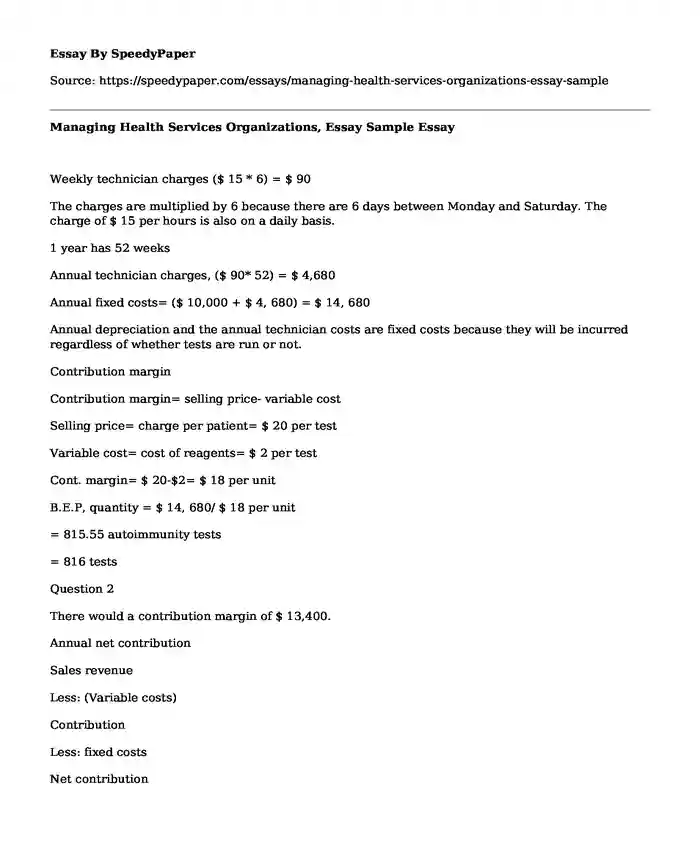# Managing Health Services Organizations, Essay Sample

Published: 2022-04-28Type of paper: Essay Categories: Financial management Pages: 2 Wordcount: 412 words
143 views

Weekly technician charges (\$ 15 * 6) = \$ 90

Is your time best spent reading someone else’s essay? Get a 100% original essay FROM A CERTIFIED WRITER!

The charges are multiplied by 6 because there are 6 days between Monday and Saturday. The charge of \$ 15 per hours is also on a daily basis.

1 year has 52 weeks

Annual technician charges, (\$ 90* 52) = \$ 4,680

Annual fixed costs= (\$ 10,000 + \$ 4, 680) = \$ 14, 680

Annual depreciation and the annual technician costs are fixed costs because they will be incurred regardless of whether tests are run or not.

Contribution margin

Contribution margin= selling price- variable cost

Selling price= charge per patient= \$ 20 per test

Variable cost= cost of reagents= \$ 2 per test

Cont. margin= \$ 20-\$2= \$ 18 per unit

B.E.P, quantity = \$ 14, 680/ \$ 18 per unit

= 815.55 autoimmunity tests

= 816 tests

Question 2

There would a contribution margin of \$ 13,400.

Annual net contribution

Sales revenue

Less: (Variable costs)

Contribution

Less: fixed costs

Net contribution

Annual sales,

5 tests a day from Monday- Saturday

5*6= 30 tests a week

1 year has 52 weeks,

30* 52= 1,560 tests a year

Sales revenue

\$ 20/ tests

Total sales revenue a year

\$20* 1,560= \$ 31,200

Variable costs

\$2/ test

Total variable costs= \$2 * 1,560= \$ 3,120

Fixed costs

Annual depreciation \$ 10,000

Annual technician charges \$ 4,680

Total sales revenue \$ 31,200

Less: total variable costs (\$ 3,120)

Contribution \$ 28,080

Less: fixed assets

Annual depreciation (\$ 10,000)

Annual technician costs (\$ 4, 680)

Net annual contribution \$ 13,400)

Question 3

Total test per year is 1,560

We assume 1 test is equal to 1 patient

Half the total patients number will be 1,560/2= 780 patients

At break even, the profit is 0

Desired profit= 0

BEP= fixed costs in total+profitmargin contributed in unit = (\$ 14, 680 + 0)/ (\$20-\$2)

=\$14,680/ \$18

=815. 55 patients

= 816 patients

The laboratory will not break even on the equipment if half of the patients have Medicare coverage (Longest, Rakich, & Darr, 2000).The number of half patients of 780 is lower than 816 which is the number of patients required to break even.

We will have to do a capital budgeting analysis of the project to see if the project is worthwhile.

Application of payback formula

Payback time = Preliminary investmentCash flow AnnuallyInitial investment is the purchase cost of the Machine which is \$ 50,000.

Annual cash flows

Sales revenue- expenses

We will use the number of patients who are not under Medicare in a year of 780

Annual Sales revenue 780* \$20= \$ 15,600

Annual Variable expenses 780* \$ 2= \$ -1, 560

\$ 14,040

Less: technician costs \$-4, 680

Total annual cash inflows \$ 9,360

Depreciation is not included in the calculation as it is a non-cash expense, no actual cash is paid.

Payback period= \$ 50,000/ \$ 9,360= 5.34 years

The payback period is 5.34 years.

The hospital should not acquire the machine because the initial cost of investment of \$ 50,000 will be recovered after 5 years which is way past the useful life of the machine of 5 years (Longest, Rakich, & Darr, 2000).

Reference

Longest, B.B., Rakich, J.S., & Darr, K. (2000). Managing health services organizations and system. Baltimore: Health Professions Press

Managing Health Services Organizations, Essay Sample. (2022, Apr 28). Retrieved from https://speedypaper.com/essays/managing-health-services-organizations-essay-sample

Request Removal

If you are the original author of this essay and no longer wish to have it published on the SpeedyPaper website, please click below to request its removal:

Liked this essay sample but need an original one?

Hire a professional with VAST experience!